Mobile QR Code1. (Department of Electronics Engineering, Hanyang University, 222 Wangshimni-ro, Seongdong-gu, Seoul 04763, Korea)

Dynamic biasing, fast transient response, output capacitor-less, low-dropout regulator, low-quiescent current

## I. INTRODUCTION

Recently, fully integrated on-chip power management integrated circuits (PMICs) have been demanded to realize the wearable devices requiring low power consumption and small form factor, while having a low output voltage of about 1 V. These low power consumption and small form factor have been achieved by reducing the bias current and the number of off-chip components, respectively, so that the battery usage time can be extended. Especially, in the low-dropout (LDO) regulator, the quiescent current can be reduced by minimizing the bias current of the amplifier. However, as the bias current decreases, the slew rate greatly decreases, resulting in a slow transient response. Therefore, it is important for the LDO regulator to achieve fast transient response, while maintaining a bias current as low as possible. In addition, to realize a small-sized LDO regulator, capacitor-less structures have been widely adopted (1-6).

To meet the above requirements, several biasing methods for the capacitor-less LDO regulators have been studied (2-6). The adaptive biasing method in (2,3) determined the bias current of the LDO regulator according to the load current. However, this LDO regulator had a large bias current at heavy load, resulting in an unnecessary current consumption. In addition, it could not maintain a sufficient bias current when the load current changed from heavy to light load. The dynamic biasing method in (4-6) instantaneously increased the bias current to improve the slew rate only when the output voltage varied significantly. The increased bias current stabilized the output voltage, but could not achieve fast transient enough. Moreover, a high pass filter including the large resistor and capacitor was used to detect the variation in the output voltage, resulting in an increase in the LDO regulator size.

In this paper, a capacitor-less LDO regulator is proposed using an advanced dynamic biasing (ADB) method in an attempt to achieve the fast transient response and low power consumption. The proposed LDO regulator efficiently detects the load transient without a large-sized high pass filter, thus achieving the fast transient response and small chip area, while maintaining a bias current low.

Section II describes the operation principle of the proposed LDO regulator with the ADB method. In Section III, the circuit implementation of the proposed transient detection circuit (TDC) and bias control circuit (BCC) are explained in detail. In Section IV, the measurement results of the proposed LDO regulator are analyzed and compared with prior works. Finally, the conclusions are given in Section V.

Fig. 1 shows the block diagram of the proposed LDO regulator using an ADB method, which includes an amplifier (AMP), the TDC, and the BCC. The output voltage of the LDO regulator ($V_{OUT}$) is regulated to [$V_{REF}$${\times}(1+R_{F1}/R_{F2})] from the input voltage (V_{IN}), where V_{REF} is a reference voltage and both R_{F1} and R_{F2} are the feedback resistors. When the load current (I_{LOAD}) varies from light to heavy load or vice versa, a voltage variation of undershoot or overshoot occurs at V_{OUT}, respectively. The TDC detects an undershoot or overshoot variation and generates a signal, V_{UN} or V_{OV}, respectively. When V_{UN} or V_{OV} occurs, the BCC increases a bias current (I_{B}) during load transients by dynamically adjusting a variable bias current (I_{V}), while maintaining I_{B} low in the steady state. In this way, the proposed ADB method can achieve fast transient and low power consumption. Fig. 1. Block diagram of the proposed LDO regulator using advanced dynamic biasing method.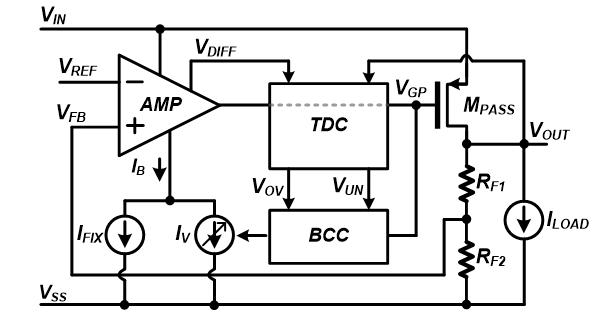Fig. 2 shows the conceptual timing diagrams of the proposed LDO regulator. When an undershoot occurs at V_{OUT} as I_{LOAD} changes from light to heavy load as shown Fig. 2(a), the TDC detects the undershoot and produces a high V_{UN}. The BCC then increases I_{B} until V_{OUT} at heavy load is stabilized. When an overshoot occurs at V_{OUT} as I_{LOAD} changes from heavy to light load as shown Fig. 2(b), the TDC detects the overshoot and produces a low V_{OV}. The BCC then increases I_{B} until V_{OUT} at light load is stabilized. Fig. 2. Conceptual timing diagrams of the proposed LDO regulator when (a) undershoot, (b) overshoot occurs at V_{OUT}.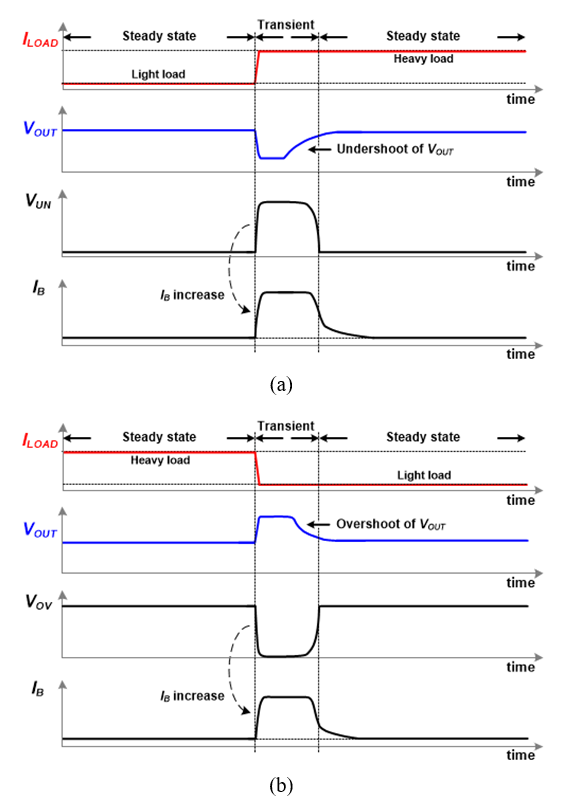## III. CIRCUIT IMPLEMENTATION Fig. 3 shows the detailed schematic of the proposed LDO regulator. The AMP employs a multi-stage cascade structure to achieve a high gain when an input voltage is low. To avoid unstable feedback loop caused by the added pole using a multi-stage, a Q-reduction technique is adopted with the use of a miller compensation capacitor (C_{Q1}) and a Q-reduction capacitor (C_{Q2}) (1). The TDC, which detects the transient responses at V_{OUT}, consists of the overshoot detection and undershoot detection circuits, both of which employ a source follower and a current comparator. The BCC, which consists of two switches, S_{1} and S_{2}, two current mirrors including M_{A5} and M_{A2}, and two MOSFETs, M_{A4} and M_{A1}, adjusts I_{V} according to I_{LOAD} during load transients. M_{A1} and M_{A4} increase the bias current, I_{V}, when the undershoot or overshoot occurs. In this work, the PMOS is used for M_{A1} to increase I_{V} by decreasing the gate voltage of M_{PASS} (V_{GP}) when the undershoot occurs. On the other hand, the NMOS is used for M_{A4} to increase I_{V} by increasing V_{GP} when the overshoot occurs. Fig. 3. Schematic of the proposed LDO regulator including TDC and BCC.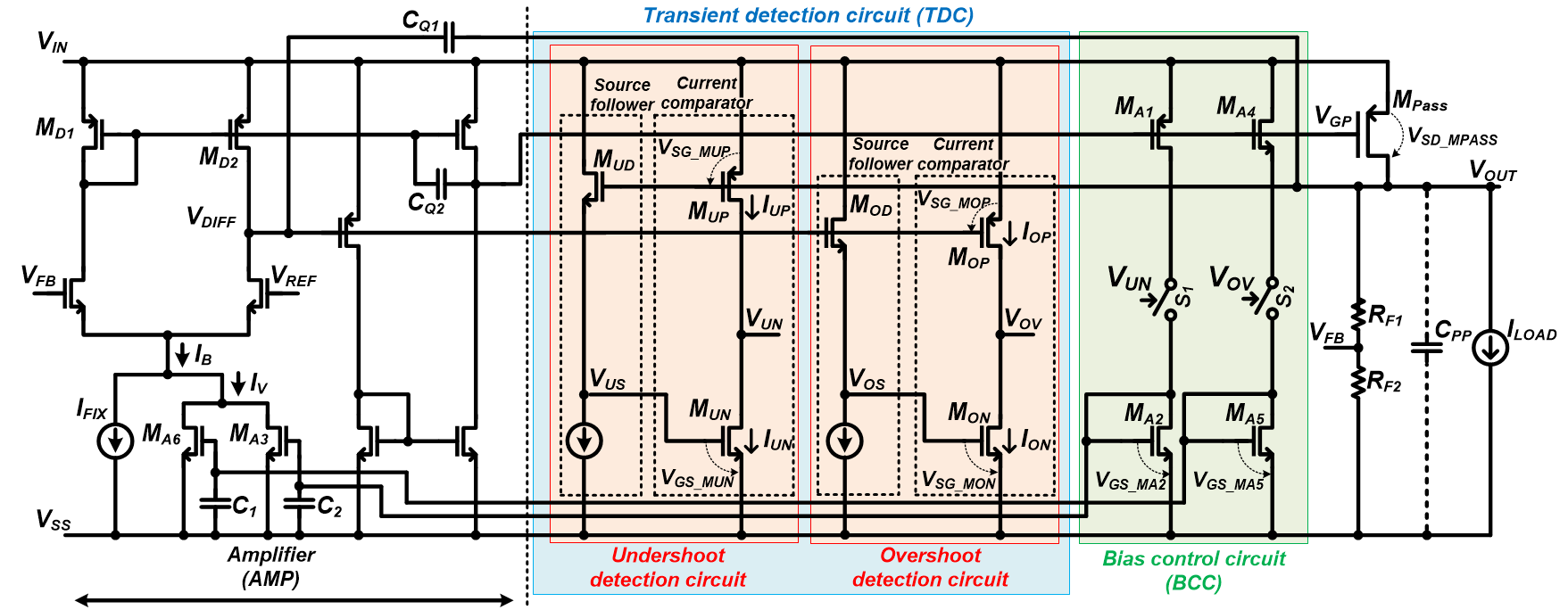As previously described, when I_{LOAD} varies from light to heavy load or vice versa, the TDC detects a voltage variation of undershoot or overshoot occurred at V_{OUT}, respectively. The BCC maintains I_{B} low during the steady state and increases I_{B} during the transient state until V_{OUT} is stabilized. The detailed operations for the undershoot and overshoot voltage variations are described next. First, the operation of the undershoot detection circuit along with the BCC is as follows. Before an undershoot occurs at V_{OUT}, I_{LOAD}, which is constantly supplied to V_{OUT} at light load, remains in the steady state. In the undershoot detection circuit, the source-drain voltage of M_{PASS} (V_{SD\_MPASS}), which is the dropout voltage of the LDO regulator, is equal to the source-gate voltage of M_{UP} (V_{SG\_MUP}). Here, V_{SD\_MPASS} is designed to be 200 mV with a PMOS threshold voltage (V_{THP}) of 550 mV. Therefore, V_{SD\_MPASS} is less than the threshold voltage of M_{UP}, V_{THP}, and thus M_{UP} remains turned off. On the other hand, the source-followed voltage of M_{UD} (V_{US}), which is [V_{OUT} - threshold voltage of M_{UD} (V_{THN})], is equal to the gate-source voltage of M_{UN} (V_{GS\_MUN}), where V_{THN} is an NMOS threshold voltage. Here, V_{OUT} is designed to be 1 V with a V_{THN} of 450 mV. Therefore, V_{US} is greater than the threshold voltage of M_{UN}, V_{THN}, and thus M_{UN} remains turned on. Consequently, V_{UN} becomes near V_{SS}, thus turning off S_{1} in the BCC, resulting in maintaining I_{B} low. When an undershoot occurs at V_{OUT} as I_{LOAD} varies from light to heavy load, V_{SG\_MUP} becomes greater than V_{GS\_MUN} because V_{OUT} decreases by the undershoot voltage at V_{OUT}, thus increasing the current flowing through M_{UP} (I_{UP}), whereas decreasing the current flowing through M_{UN} (I_{UN}). Therefore, V_{DS} of M_{UP} becomes less than V_{DS} of M_{UN} to equalize the current levels of M_{UN} and M_{UP}, thus decreasing V_{UN} to V_{IN} and turning on S_{1} in the BCC. Consequently, the gate-source voltage of M_{A2} (V_{GS\_MA2}) increases, thus increasing the current flowing through M_{A3}, resulting in an increase in I_{V} of the AMP until V_{OUT} at heavy load is stabilized. Second, the operation of the overshoot detection circuit along with the BCC is as follows. Before an overshoot occurs at V_{OUT}, I_{LOAD}, which is constantly supplied to V_{OUT} at heavy load, remains in the steady state. In the overshoot detection circuit, the source-gate voltage of M_{OP} (V_{SG\_MOP}), which is [V_{IN} - V_{DIFF}], is greater than the threshold voltage of M_{OP}, V_{THP}, because [V_{IN} - V_{DIFF}] is slightly greater than V_{THP} due to a diode-connected M_{D1}. Here, V_{DIFF} is a biased voltage of the AMP when the LDO regulator is in the steady state, where, V_{IN} is designed to be 1.2 V with a V_{THP} of 550 mV. Consequently, M_{OP} remains turned on. On the other hand, the source-followed voltage of M_{OD} (V_{OS}), which is [V_{IN} - threshold voltage of M_{D1} (V_{THP})- threshold voltage of M_{OD} (V_{THN})], is equal to the gate-source voltage of M_{ON} (V_{GS\_MON}). Here, V_{THN} is 450 mV. Therefore, V_{OS} is less than the threshold voltage of M_{ON}, V_{THN}, and thus M_{ON} remains turned off. Consequently, V_{OV} becomes near V_{IN}, thus turning off S_{2} in the BCC, resulting in maintaining I_{B} low. When an overshoot occurs at V_{OUT} as I_{LOAD} varies from heavy to light load, V_{SG\_MOP} becomes less than V_{GS\_MON} because V_{OUT} increases by the overshoot voltage at V_{OUT}, thus decreasing the current flowing through M_{OP} (I_{OP}), whereas increasing the current flowing through M_{ON} (I_{ON}). Therefore, V_{DS} of M_{ON} becomes less than V_{DS} of M_{OP} to equalize the current levels of M_{ON} and M_{OP}, thus decreasing V_{OV} to V_{SS} and turning on S_{2} in the BCC. Accordingly, the gate-source voltage of M_{A5} (V_{GS\_MA5}) increases, thus increasing the current flowing through M_{A6}, resulting in an increase in I_{V} of the AMP until V_{OUT} at light load is stabilized. As I_{V} increases during the transition of the undershoot and overshoot, I_{B} increases, and thereby the gate voltage of M_{PASS} is rapidly changed to achieve a fast transient response. In addition, since I_{B} remains low during the steady states, the power consumption can be minimized. Capacitors of C_{1} and C_{2} are used to prevent abrupt switching. When S_{1} and S_{2} are turned off, M_{A5} and M_{A2} operate in the sub-threshold region and slowly discharge C_{1} and C_{2}. Thus, I_{B} gradually decreases, and thus variations in V_{OUT} caused by an abrupt change in I_{B} can be minimized. Fig. 4 shows the AC simulation results of the proposed LDO regulator, showing that the frequency response and phase margin are 152 kHz and 72 degrees at I_{LOAD} = 100~mA, and 211 kHz and 77 degrees at I_{LOAD} = 100 {\mu}A, respectively. Fig. 4. AC Simulation results of the proposed LDO regulator; (a) frequency response, (b) phase margin.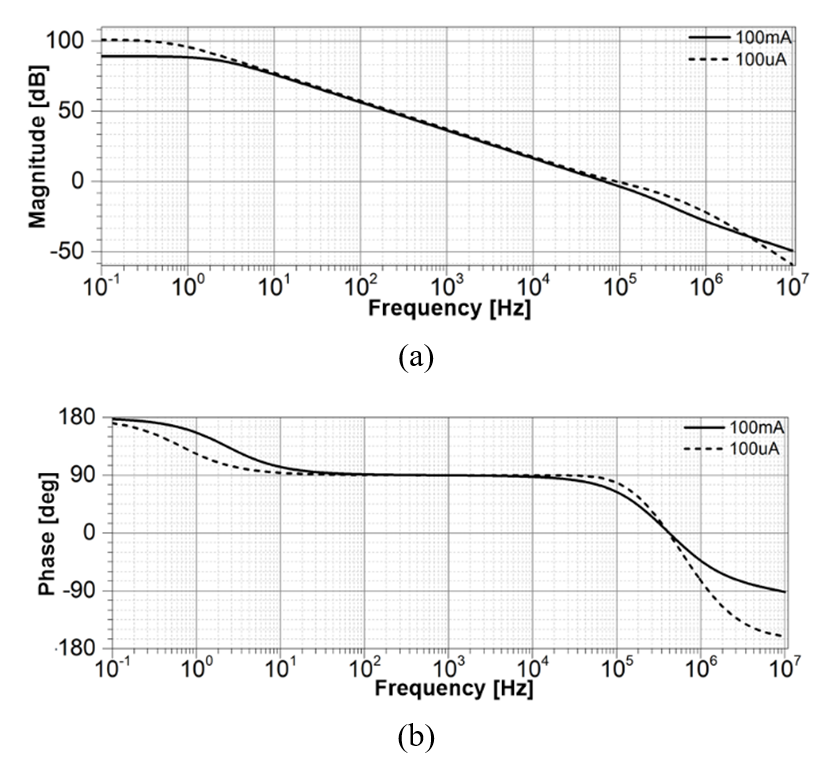## IV. MEASUREMENT RESULTS Fig. 5 shows the chip photomicrograph of the proposed LDO regulator, which was fabricated using a 0.18-{\mu}m CMOS process and occupied an area of 0.05~mm^{2} including dummy cells. In the measurement, the output capacitance of the proposed LDO regulator, which is a parasitic capacitance of the metal line, was modelled as C_{PP} (1). The transient response of the proposed LDO regulator was measured using an off-chip C_{PP} of 100 pF and compared with those achieved in previous works. Fig. 6 shows the measured load transient responses of the proposed LDO regulator at a V_{IN} of 1.2 V and a [V_{IN} - V_{OUT}] of 200 mV, when I_{LOAD} varies between 100 {\mu}A and 100 mA. In addition, both the rising and falling times of I_{LOAD} were given to be 0.5 {\mu}s. The measurement results showed that V_{OUT} was stabilized to 99\% of a target voltage (1 V) within 0.6 {\mu}s and 1.2 {\mu}s when I_{LOAD} changes from light to heavy load and vice versa, respectively. Fig. 6. (a) Measured load transient response, and zoomed-in responses, (b) from light to heavy load, (c) from heavy to light load.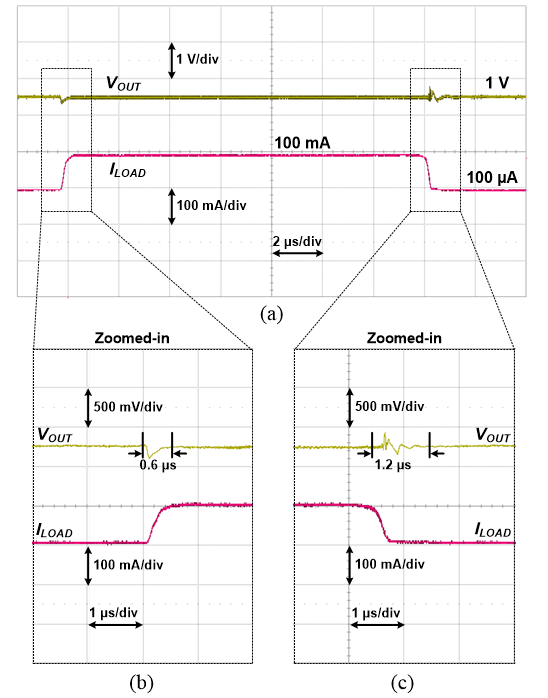The quiescent current (I_{Q}) was measured to be 2.0 {\mu}A at an I_{LOAD} of 100 {\mu}A, which was obtained by subtracting I_{LOAD} from the input current. Under these conditions, the undershoot and overshoot voltages were measured to be 215 mV and 288 mV, respectively. Table 1 shows the performance comparison between the proposed LDO regulator and prior works. The proposed LDO regulator showed the best figure-of-merit (FOM), where FOM is [T_{settle} {\times}$$I_{Q}$/$I_{LOAD(MAX)}$] (2) compared to prior works.

Table 1. Performance comparison with prior works

     This work Process technology (nm) 130 350 180 130 180 $I_{\mathrm{LOAD(MAX)}}$ (mA) 100 100 50 50 100 $V_{\mathrm{in}}$ (V) 1.0 1.5 1.9 0.9 1.2 $V_{\mathrm{out}}$ (V) 0.8 1.3 1.8 0.8 1.0 $I_{\mathrm{Q}}$ (${\mu}$A) 2.9 26.0 7.0 1.3 2.0 $C_{\mathrm{LOAD}}$ (pF) 100 100 100 100 100 $T_{\mathrm{settle}}$ (${\mu}$S) 1.7 0.5 3.9 28.0 1.2 FOM$^{\mathrm{1)}}$(ns) 0.049 0.130 0.546 0.728 0.024

$^{\mathrm{1)}}$Figure-of-merit was calculated by $T_{settle}$ ${\times}$$I_{Q}$$/I_{LOAD(MAX)}$.

## V. CONCLUSIONS

This paper proposes a fast transient and low power capacitor-less LDO regulator with an ADB method. The proposed LDO regulator improved the load transient response and achieved a quiescent current of 2.0 ${\mu}$A at light load. When the load current changes from light to heavy load and vice versa, the output voltage was settled within 0.6 ${\mu}$s and 1.2 ${\mu}$s, respectively, representing that a fast transient response was achieved while maintaining a low power. Therefore, the proposed LDO regulator using an ADB method is suitable for wearable applications requiring fast transient response and low power consumption.

### REFERENCES

1
Sai Kit Lau , Philip K. T. Mok , Ka Nang Leung , Mar., 2007, A low-dropout regulator for SoC with q-reduction, Solid-State Circuits, IEEE Journal of, Vol. 42, No. 3, pp. 658-6642
Zhan Chenchang, Ki Wing-Hung, May., 2010, Output-capacitor-free adaptive biased low-dropout regulator for system-on-chips, Circuits Systems I: Fundamental Theory and Applica¬tions, IEEE Transactions on, Vol. 57, No. 5, pp. 1017-10283
Qu Xi, Zhou Ze-Kun, Zhang Bo, Aug., 2014, Ultralow-power fast-transient output-capacitor-less low-dropout regulator with advanced adaptive biasing circuit, Devices & Systems, IET Journal of, Vol. 9, No. 3, pp. 172-1804
Wu C. -H., Chang-Chien L. -R., Feb., 2012, Design of the output-capacitorless low-dropout regulator for nano-second transient response, Power Elec-tronics, IET Journal of, Vol. 5, No. 8, pp. 1551-15595
Perez-Bailion J., Marquez A., Calvo B., Merdrano N., Martinez P.A., Apr., 2017, Fast-transient high-performance 0.18 μm CMOS LDO for battery-powered systems, Electronics Letters, IET Journal of, Vol. 53, No. 8, pp. 551-5526
Ho Marco, Leung Ka Nang, Mar., 2011, Dynamic bias-current boosting technique for ultralow-power low-dropout regulator in biomedical applications, Circuits and Systems II, IEEE Transactions on, Vol. 58, No. 3, pp. 174-178## Author

Jae-Hoon Jung received an M.S degree in Electronics and Computer Engineering from Hanyang Univer-sity, Seoul, Korea, in 2018. He is currently with Silicon Mitus Com-pany Inc., Seongnam, South Korea, where he has been involved in the design of the power management ICs.

Jae-Hyung Jung received B.S. degrees in Electrical and Computer Engineering from Hanyang Univer-sity, Seoul, Korea, in 2012. He is currently pursuing a Ph. D. at the same university. His research interests low-power CMOS mixed circuit design, and energy harvesting system.

Young-Ho Jung received a Ph. D. degree in Electrical and Computer Engineering from Hanyang Univer-sity, Seoul, Korea, in 2018. He is currently with Samsung Electronics Company Ltd., Hwasung, South Korea, where he has been involved in the design of various switching mode power converters for smart mobile devices.

Hoe-Eung Jeong received a. B.S. degree in electronics and Computer Engineering from Hanyang Univer-sity, Seoul, Korea, in 2011, and a M.S. degree in electronics science and technology from Tsinghua University, Beijing, China, in 2014. He is currently pursuing a Ph. D. degree in electronics and computer engineering at Hanyang University. His research interests include low-power power management converter design for mobile, wearable, medical, IoT devices, and so on.

Seong-Kwan Hong received a Ph. D. degree in electrical engineering from Georgia Institute of Technology, Atlanta, GA, USA, in 1994. He is currently a Research Professor at Hanyang University, Seoul, Korea.

Oh-Kyong Kwon received a Ph. D. degree in electrical engineering from Stanford University, Stanford, CA, USA, in 1988. He is currently an HYU Distinguished Professor with the Department of Electronic Engineering, Hanyang University, Seoul, Korea.